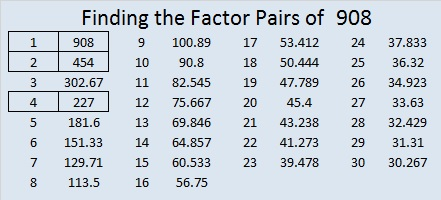# 908 Haunted House

I combined most of this week’s puzzles to make a haunted house. None of the levels are listed on it, but I will include the levels as I publish each puzzle separately sometime this week. If you enter this haunted house, will you be able to escape? Warning: This should not be the first Find The Factors puzzle you try. A couple of the puzzles in it may be easy, but the rest could be very scary!Print the puzzles or type the solution on this excel file: 12 factors 905-913

908 can be written as the sum of two consecutive odd numbers:

• 453 + 455 = 908

Four consecutive even numbers:

• 224 + 226 + 228 + 230 = 908

And eight consecutive counting numbers:

• 110 + 111 + 112 + 113 + 114 + 115 + 116 + 117 = 908

There wasn’t much to say about the number 908, but it’s always possible to find something.

• 908 is a composite number.
• Prime factorization: 908 = 2 × 2 × 227, which can be written 908 = 2² × 227
• The exponents in the prime factorization are 2 and 1. Adding one to each and multiplying we get (2 + 1)(1 + 1) = 3 × 2  = 6. Therefore 908 has exactly 6 factors.
• Factors of 908: 1, 2, 4, 227, 454, 908
• Factor pairs: 908 = 1 × 908, 2 × 454, or 4 × 227
• Taking the factor pair with the largest square number factor, we get √908 = (√4)(√227) = 2√227 ≈ 30.1330383